# Learning to Convert Decimals Into Fractions [Tutorial]This tricky concept has stumped plenty of math students, but you won’t be one of them after this simple tutorial!

In this edition of Math with Margo, convert decimals into fractions (or ratios) with ease in her step-by-step practice problem.

Let’s do this!

## How to turn decimals into fractions

Problem: How is the decimal .32 represented as a fraction?#### Step One: Set up the problem and multiply the fraction by 1

Let’s try to convert a decimal to a fraction.

First, we will put our decimal in fraction form by placing it over 1. Any value divided by 1 is itself, so we are not changing its value, just the look.

Similarly, any value multiplied by 1 is itself, so we can multiply our fraction by a giant 1 and not change the value of the fraction.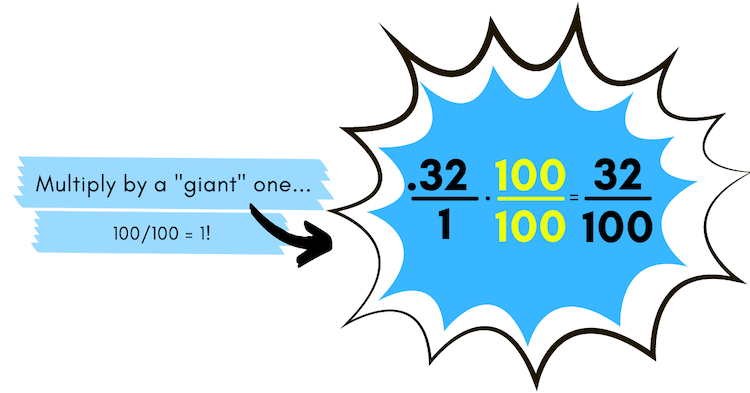#### Step Two: Simplify the fraction

Now you have your decimal in fraction form, but we need to check if it can be simplified.

2 can go into 32 and 100 evenly, and 2/2 is the equivalent of 1, so we are left with 16/50.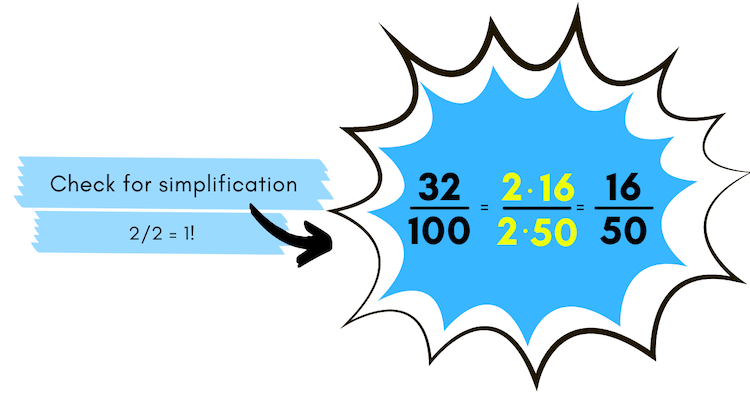Similarly, 2 can go into 16 and 50 evenly, so we are left with 8/25. No numbers go into both 8 and 25 evenly, so this is the answer in simplest form.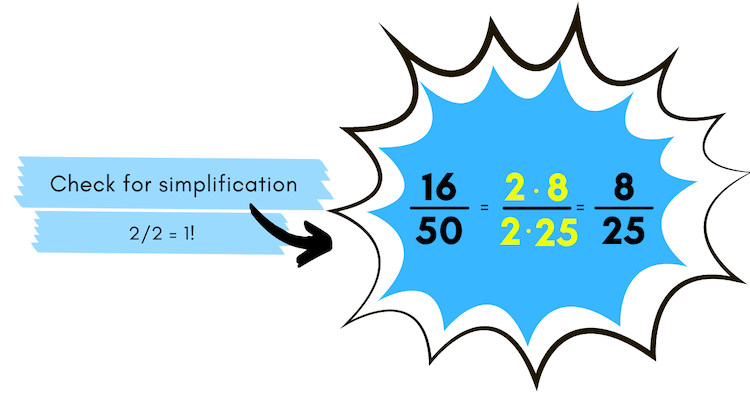#### Step Three: Check your work

Therefore, .32 = 8/25. This seems logical since .32 is close to ⅓ and visually 8/25 looks like one third.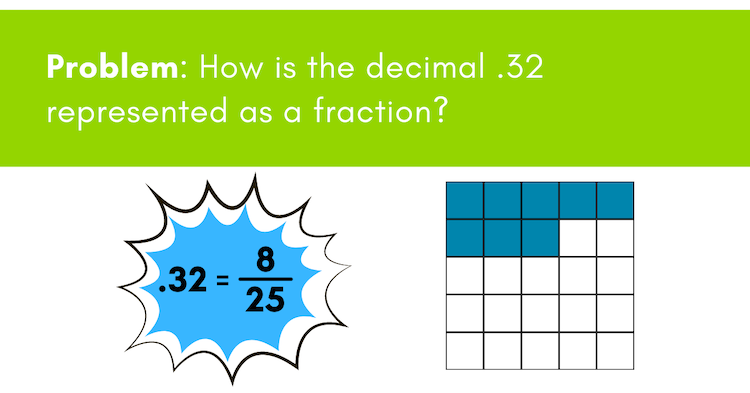#### Step Four: Double check your work by comparing the fraction with the decimal

The fraction bar is also known as division, so this can be read as 8 divided by 25.#### Step Five: Divide to reach final answer

Since 25 does not go into 8, we must add a decimal and a 0 after 8. 8 and 8.0 are equivalent—just like saying \$8 or \$8.00, they are both equal in value. You can add a decimal and as many 0s, and it still will not change its value.

Now ask yourself, how many times does 25 go into 80? 3 is correct, since 25 times 3 equals 75, which is as close as you can get to 80 without going over.

Make sure you bring the decimal straight up.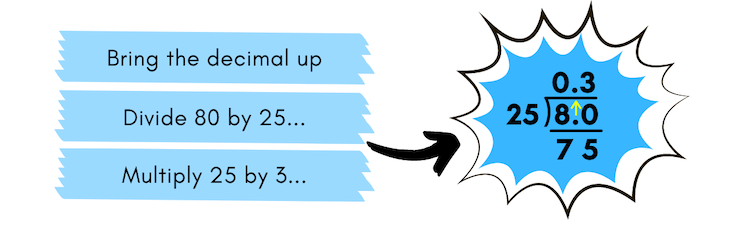Learn more: Easy Division Tricks & Tips

Since we have a remainder of 5, we need to add another 0 to the end of 8.0 and bring the 0 down.

Now, ask yourself, how many times does 25 go into 50? 2 is correct, since 25 times 2 equals 50.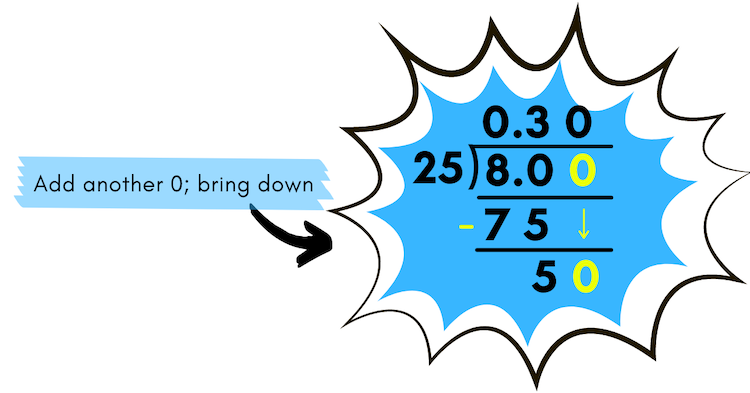####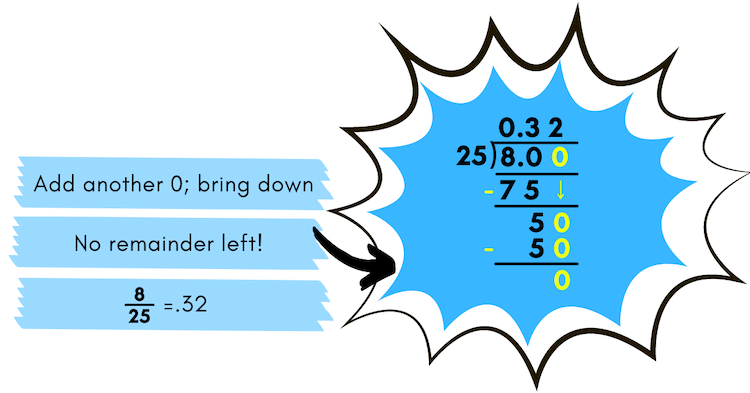Since we no longer have a remainder, we are done! Your final answer is 8/25 = 0.32.

Congratulations! You did it. Wondering how to convert fractions into decimals? We’ve got you covered in this related post.

And there you have it! Another math concept mastered with a little help from Margo. For more math resources, check out our solve for x tutorial and online math tutoring opportunitiesVirginia started with iD Tech at the University of Denver in 2015 and has loved every minute since then! A former teacher by trade, she has a master's in education and loves working to embolden the next generation through STEM. Outside the office, you can usually find her reading a good book, struggling on a yoga mat, or exploring the Rocky Mountains.

Featured Posts

Categories

Authors